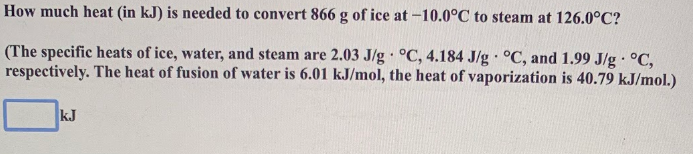# How much heat (in kJ) is needed to convert 886 g of ice at -10.0 degrees C to steam at 126.0 degrees C? (The specific heats of ice, water, and steam are 2.03 J/g. degrees C, 4.184 J/g. degrees C, and 1.99 J/g. degrees C, respectively. The heat of fusion of water is 6.01 kJ/mol, the heat of vaporization is 40.79 kJ/mol.)